# Shoes and slippers

Shoes were three times as many as slippers. If shoes were cheaper by 120, it would be twice as expensive as slippers. How much cost shoes and how much slippers?

Correct result:

x =  360 Kc
y =  120 Kc

#### Solution:

x= 3y
x-120 = 2y

x= 3•y
x-120 = 2•y

x-3y = 0
x-2y = 120

x = 360
y = 120

Our linear equations calculator calculates it.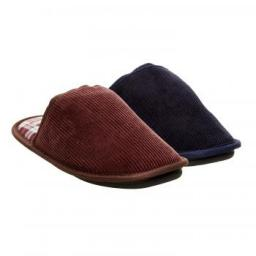We would be pleased if you find an error in the word problem, spelling mistakes, or inaccuracies and send it to us. Thank you!Tips to related online calculators
Do you have a linear equation or system of equations and looking for its solution? Or do you have quadratic equation?

## Next similar math problems:

• Three workersThree workers were rewarded CZK 9200 and the money divided by the work they have done. First worker to get twice than the second, the second three times more than the third. How much money each worker received?
• Tea blendTea blends are maked from two kinds of tea. In standard tea mixture are two teas in the ratio 1:3 and 40 g costs 42 CZK. In the premium tea mixture are weighing two teas in the ratio 1:1 and 50 grams costs 60 CZK. How much cost 10 grams of more expensive
• PropertyThe length of the rectangle-shaped property is 8 meters less than three times of the width. If we increase the width 5% of a length and lendth reduce by 14% of the width it will increase the property perimeter by 13 meters. How much will the property cost
• Chickens and rabbitsIn the yard were chickens and rabbits. Together they had 27 heads and 86 legs. How many chickens and how many rabbits were in the yard?
• Euler problem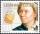Someone buys a 180 tolars towels. If it was for the same money of 3 more towels, it would be 3 tolars cheaper each. How many were towels?
• Mother and daughter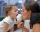Three years ago mother was three times older than daughter. After nine years she will be only twice old. How old is mother (and daughter)?
• Purchase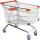Mother bought 5 boxes of milk and 7 kg of potatoes and paid a total CZK 147. Aunt bought 7 boxes of milk and 3 kg of potatoes and paid 131 CZK. What is the price of one carton of milk and 1 kg of potatoes? How CZK together would have saved if bought at th
• WineA bottle of wine costs 21 euros; and wine is 20 times more expensive than a bottle. How much a bottle cost?
• Jane and Miro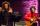Jane brother Miro is 42 years. And he is three times old as it was Jane when Miro was for so many years as there are now Jane. How old is Jane?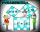Two fifth-graders teams competing in math competitions - in Mathematical Olympiad and Pytagoriade. Of the 33 students competed in at least one of the contest 22 students. Students who competed only in Pytagoriade was twice more than those who just compete
• Three friends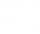Peter is eight times older than Rado, Rado is 3 times younger than Joseph. Together they have 120 years. Determine how many are each of them.
• Bottle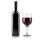Bottle with wine costs 58 USD. Wine is 27 USD more expensive than the empty bottle. How much is the bottle itself?
• Division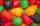Three siblings Helena, Oliver and George split the bag with candies on merit in the ratio 6:1:4. How many candies should each get if in bag were 88?
• Sweets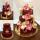3 chocolate and 7 cakes cost 85, - CZK. 2 chocolates and 6 cakes cost 86, - CZK. How much is 5 chocolates and 9 cakes? I wonder how to get the result, but only by logic without the use of a system of equations
• Tippler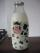Bottle with cork cost 8.8 Eur. The bottle is 0.8 euros more expensive than cork. How much is a bottle and the cork?
• Barbara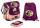Barborka goes to school with backpack that was 2 - times more expensive than a bag slipper. If backpack was 36 euros cheaper it was cost same as bag slipper. How many cost backpack and how many bas slipper?
• Scouts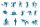Three scouts went on a three day trip. The second day went 4 km more than the first day. The third day went two times less than the first day. They went along 54 km. How many kilometers went every day together?